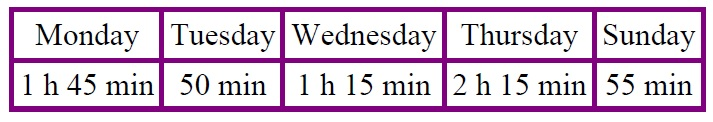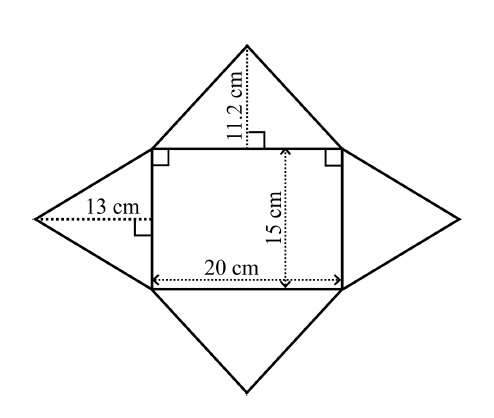# Maths Quiz 2019/2020

This quiz has been designed as a fun activity and comprises of 12 questions covering a range of maths questions.

We hope you enjoy the quiz
1. The information provided in this quiz will only be shared with our partner organisations (for full details https://urtuulr.com/privacy-policy/).

I have read and understood the above

Please tick to accept to continue with this quiz *This question is required.
Contact information
(this will allow us to send you your goodie bag, if you are successful) *This question is required.
Mary picked 5 apples and Bill picked 9. The children shared all of their apples evenly. How many did each child get? *This question is required.
Correct!

5 + 9 = 14 They each got seven apples
Sorry, incorrect.

5 + 9 = 14 They each got seven apples
Bill bought an eraser that cost 85p. He paid with £1.
What was his change? *This question is required.
Correct!

His change was 15p
Sorry, incorrect

His change was 15p
Solve: 5 × 25 *This question is required.
Correct!

5 × 25 = 125. You can solve it by adding repeatedly: 25 + 25 + 25 + 25 + 25 = 125
Sorry, incorrect

5 × 25 = 125. You can solve it by adding repeatedly: 25 + 25 + 25 + 25 + 25 = 125
The TV show starts at 6:25 PM and ends at 7:10 PM.
How long is it? *This question is required.
Correct!

45 minutes
Sorry, incorrect

45 minutes
Luis kept track of how long it took him to do his homework:
How much time did he spend with homework in total?*This question is required.
Correct!

1 h 45 min + 50 min + 1 h 15 min + 2 h 15 min + 55 min = 4 h 180 min, which is 7 hours.
Sorry, incorrect

1 h 45 min + 50 min + 1 h 15 min + 2 h 15 min + 55 min = 4 h 180 min, which is 7 hours.
Joe had saved £264. He spent 3/8 of that to buy a camera.
How much did the camera cost? *This question is required.
Correct!

It cost £99. First find 1/8 of £264: £264 ÷ 8 = £33. Then to find 3/8 of it, multiply 3 × £33 = £99.
Sorry, incorrect

It cost £99. First find 1/8 of £264: £264 ÷ 8 = £33. Then to find 3/8 of it, multiply 3 × £33 = £99.
You need 2 3/4 cups of flour for one batch of rolls.
How much flour would you need for three batches of rolls. *This question is required.
Correct!

You would need 3 × (2 3/4) = 8 1/4 cups of flour to make three batches of rolls.
Sorry, incorrect

You would need 3 × (2 3/4) = 8 1/4 cups of flour to make three batches of rolls.
How many 1/4 ft pieces can you cut from a string that is 15 feet long? *This question is required.
Correct!

You can cut 60 pieces: 15 ft ÷ (1/4 ft) = 60.
Sorry, incorrect

You can cut 60 pieces: 15 ft ÷ (1/4 ft) = 60.
What is the difference between the two temperatures.

−13°C and 10°C *This question is required.
Correct!

The difference is 23 degrees
Sorry, incorrect

The difference is 23 degrees
What its surface area of the following shape.*This question is required.
Correct!

The rectangle has the area of 300 cm2. The top and bottom triangles: 2 × 20 cm × 11.2 cm ÷ 2 = 224 cm2.
The left and right triangles: 2 × 15 cm × 13 cm ÷ 2 = 195 cm2. The total surface area is 719 cm2.
Sorry, incorrect

The rectangle has the area of 300 cm2. The top and bottom triangles: 2 × 20 cm × 11.2 cm ÷ 2 = 224 cm2.
The left and right triangles: 2 × 15 cm × 13 cm ÷ 2 = 195 cm2. The total surface area is 719 cm2.
What is 0.1748 as a fraction *This question is required.
Correct!

1748/10,000
Sorry, incorrect

1748/10,000
Sally deposits £2,500 at 8% interest for 3 years.

How much can she withdraw at the end of that period? *This question is required.
Correct!

She can withdraw
£2,500 / 100 =  £25 x 8 x 3 = £600+ £2,500 = £3,100.
Sorry, incorrect

She can withdraw
£2,500 / 100 =  £25 x 8 x 3 = £600+ £2,500 = £3,100.